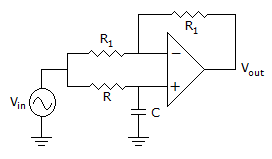# Electronics and Communication Engineering - Exam Questions Papers

1.

Compared to a p-n junction with NA = ND = 1014/cm3, which one of the following statements is TRUE for a p-n junction with NA = ND = 1020/cm3?

 A. Reverse breakdown voltage is lower and depletion capacitance is lower B. Reverse breakdown voltage is higher and depletion capacitance is lower C. Reverse breakdown voltage is lower and depletion capacitance is higher D. Reverse breakdown voltage is higher and depletion capacitance is higher

Explanation:

No answer description available for this question. Let us discuss.

2.

Which of the following are universal gates?

1. NAND
2. NOR
3. XOR
Select the correct answer from the codes given below:

 A. 1 and 2 only B. 1 and 3 only C. 2 and 3 only D. 1, 2 and 3

Explanation:

No answer description available for this question. Let us discuss.

3.

Consider the following statements:
To draw a.c. equivalent circuit of a transistors, all

1. d.c. sources are shorted
2. a.c. sources are shorted
3. d.c. sources are opened
4. a.c. sources are connected to d.c. sources
Which of the above statements is/are correct?

 A. 2 and 4 B. 1 and 2 C. 1 only D. 3 and 4

Explanation:

No answer description available for this question. Let us discuss.

4.

How many number of branches the root loci of the equation s(s + 2)(s + 3) + K(s + 2) = 0 have?

 A. Zero B. One C. Two D. Three

Explanation:

No answer description available for this question. Let us discuss.

5.

For the circuit of the following figure, with an ideal op-amp, the maximum phase shift of the output with reference to the input is :A. 0° B. 90° C. -90° D. ± 180°